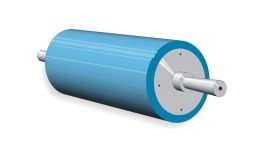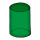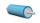# Shell area cy

The cylinder has a shell content of 300 cm square, while the height of the cylinder is 12 cm. Calculate the volume of this cylinder.

V =  596.831 cm3

### Step-by-step explanation:Did you find an error or inaccuracy? Feel free to write us. Thank you!Tips to related online calculators
Looking for help with calculating roots of a quadratic equation?
Do you have a linear equation or system of equations and looking for its solution? Or do you have a quadratic equation?
Tip: Our volume units converter will help you with the conversion of volume units.

## Related math problems and questions:

• The cylinderThe cylinder has a surface area of 300 square meters, while the cylinder's height is 12 m. Calculate the volume of this cylinder.
• The cylinderIn a rotating cylinder it is given: the surface of the shell (without bases) S = 96 cm2 and the volume V = 192 cm cubic. Calculate the radius and height of this cylinder.
• Surface of the cylinderCalculate the surface of the cylinder for which the shell area is Spl = 20 cm2 and the height v = 3.5 cm
• Diameter = heightThe cylinder's surface, the height of which is equal to the diameter of the base, is 4239 cm square. Calculate the cylinder volume.
• Rotary cylinderIn the rotary cylinder it is given: surface S = 96 cm2 and volume V = 192 cm cubic. Calculate its radius and height.
• Equilateral cylinderEquilateral cylinder (height = base diameter; h = 2r) has a volume of V = 199 cm3 . Calculate the surface area of the cylinder.
• The surfaceThe surface of the cylinder is 1570 cm2, its height is 15 cm. Find its volume and radius of the base.
• What isWhat is the height of a cylinder whose surface size is 602.88 cm2 and the content of its shell is 376.8 cm2?
• Volume and surfaceCalculate the volume and surface area of the cylinder when the cylinder height and base diameter is in a ratio of 3:4 and the area of the cylinder jacket is 24 dm2.
• Four prismsQuestion No. 1: The prism has the dimensions a = 2.5 cm, b = 100 mm, c = 12 cm. What is its volume? a) 3000 cm2 b) 300 cm2 c) 3000 cm3 d) 300 cm3 Question No.2: The prism base is a rhombus with a side length of 30 cm and a height of 27 cm. The height of t
• Surface areaThe volume of a cone is 1000 cm3 and the content area of the axis cut is 100 cm2. Calculate the surface area of the cone.
• Hexagonal prism 2The regular hexagonal prism has a surface of 140 cm2 and height of 5 cm. Calculate its volume.
• Height as diameter of baseThe rotary cylinder has a height equal to the base diameter and a surface of 471 cm2. Calculate the volume of a cylinder.
• Cylinder - hThe cylinder volume is 140 cm3. The base radius is 7 cm. Calculate the height of the cylinder.
• CylinderThe cylinder surface is 922 dm2. Its height is equal to the radius of the base. Calculate the height of this cylinder.
• Cuboid and eq2Calculate the volume of cuboid with square base and height 6 cm if the surface area is 48 cm2.
• Cuboid wallsCalculate the cuboid volume if its different walls have an area of 195cm², 135cm², and 117cm².